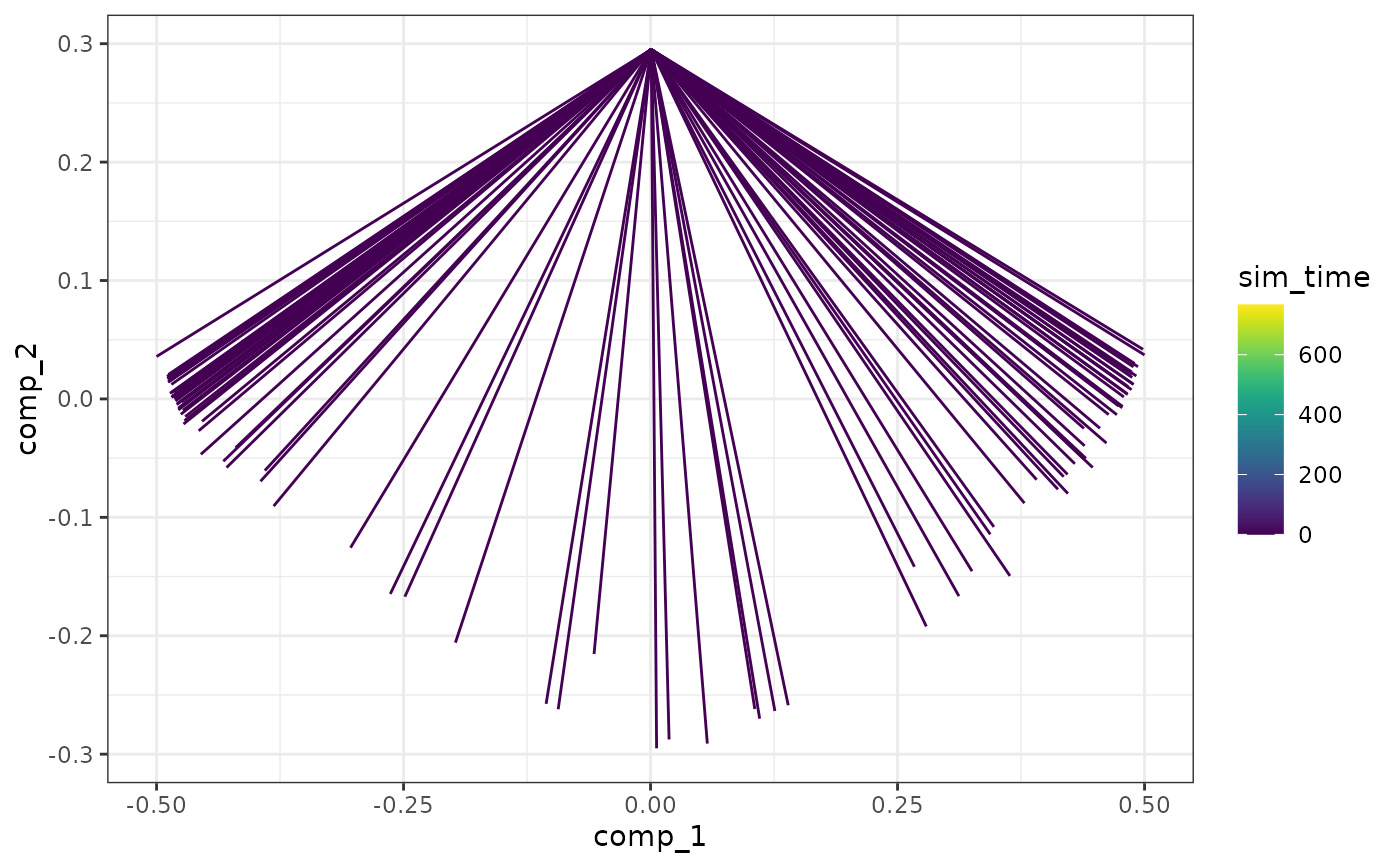Visualise the simulations using the dimred

plot_simulations(model, mapping = aes_string("comp_1", "comp_2"))

## Arguments

model A dyngen intermediary model for which the simulations have been run with generate_cells(). Which components to plot.

## Value

A ggplot2 object.

## Examples

# \donttest{
data("example_model")
plot_simulations(example_model)# }